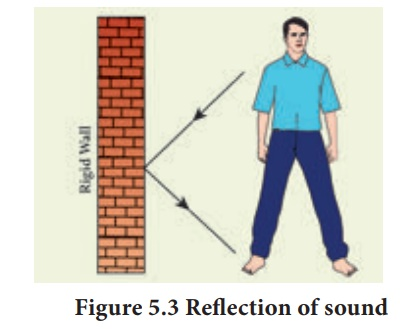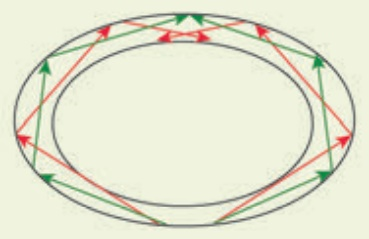Home | | Science 10th Std | Reflection of Sound

# Reflection of Sound

When you speak in an empty room, you hear a soft repetition of your voice. This is nothing but the reflection of the sound waves that you produce. Let us discuss about the reflection of sound in detail through the following activity.

REFLECTION OF SOUND

When you speak in an empty room, you hear a soft repetition of your voice. This is nothing but the reflection of the sound waves that you produce. Let us discuss about the reflection of sound in detail through the following activity.

When sound waves travel in a given medium and strike the surface of another medium, they can be bounced back into the first medium. This phenomenon is known as reflection. In simple the reflection and refraction of sound is actually similar to the reflection of light. Thus, the bouncing of sound waves from the interface between two media is termed as the reflection of sound. The waves that strike the interface are termed as the incident wave and the waves that bounce back are termed as the reflected waves, as shown in Figure 5.3## 1. Laws ofreflection

Like light waves, sound waves also obey some fundamental laws of reflection. The following two laws of reflection are applicable to sound waves as well.

·                 The incident wave, the normal to the reflecting surface and the reflected wave at the point of incidence lie in the same plane.

·                 The angle of incidencei is equal to the angle of reflection r.

These laws can be observed from Figure 5.4.In the above Figure 5.4, the sound waves that travel towards the reflecting surface are called the incident waves. The sound waves bouncing back from the reflecting surface are called reflected waves. For all practical purposes, the point of incidence and the point of reflection is the same point on the reflecting surface.

A perpendicular line drawn at the point of incidence is called the normal. The angle which the incident sound wave makes with the normal is called the angle of incidence, ‘i’. The angle which the reflected wave makes with the normal is called the angle of reflection, ‘r’.

## 2.Reflection at the boundary of a denser medium

A longitudinal wave travels in a medium in the form of compressions and rarefactions. Suppose a compression travelling in air from left to right reaches a rigid wall. The compression exerts a force F on the rigid wall. In turn, the wall exerts an equal and opposite reaction R = – F on the air molecules. This results in a compression near the rigid wall. Thus, a compression travelling towards the rigid wall is reflected back as a compression. That is the direction of compression is reversed (Figure 5.5).## 3.Reflection at the boundaryof a rarer medium

Consider a wave travelling in a solid medium striking on the interface between the solid and the air. The compression exerts a force F on the surface of the rarer medium. As a rarer medium has smaller resistance for any deformation, the surface of separation is pushed backwards (Figure 5.6). As the particles of the rarer medium are free to move, a rarefaction is produced at the interface. Thus, a compression is reflected as a rarefaction and a rarefaction travels from right to left.## 4.Reflection of sound in plane and curved surfacesWhen sound waves are reflected from a plane surface, the reflected waves travel in a direction, according to the law of reflection. The intensity of the reflected wave is neither decreased nor increased. But, when the sound waves are reflected from the curved surfaces, the intensity of the reflected waves is changed. When reflected from a convex surface, the reflected waves are diverged out and the intensity is decreased. When sound is reflected from a concave surface, the reflected waves are converged and focused at a point. So the intensity of reflected waves is concentrated at a point. Parabolic surfaces are used when it is required to focus the sound at a particular point. Hence, many halls are designed with parabolic reflecting surfaces. In elliptical surfaces, sound from one focus will always be reflected to the other focus, no matter where it strikes the wall.

This principle is used in designing whispering halls. In a whispering hall, the speech of a person standing in one focus can be heard clearly by a listener standing at the other focus.Study Material, Lecturing Notes, Assignment, Reference, Wiki description explanation, brief detail
10th Science : Chapter 5 : Acoustics : Reflection of Sound |